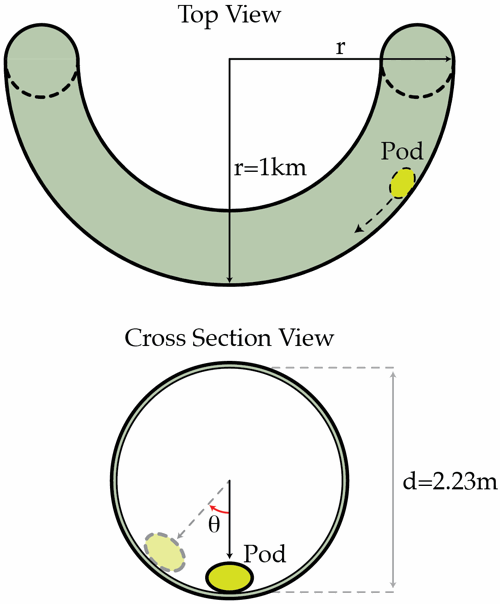# Hyperloop: CurvesThe Hyperloop is a hypothetical new fast transport system between cities, which works by launching pods that carry people through a very low air pressure tunnel. Consider a circular tunnel of diameter $2.23~\mbox{m}$ and a simple model of the Hyperloop pod as a point mass that usually travels at the bottom of the tunnel when it is straight. Tunnels can't always be straight, of course, so let us suppose that one section of the tunnel has a curve corresponding to an arc of a circle with radius $1~\mbox{km}$.

What angle in degrees does the line drawn from the center of the tunnel to the pod make with the vertical axis if the pod is traveling at a constant $300~\mbox{m/s}$ along this curved section of the tunnel?

Details and assumptions

• The acceleration of gravity is $-9.8~\mbox{m/s}^2$.
• Ignore friction as the friction force on the Hyperloop is minimal.
×

Problem Loading...

Note Loading...

Set Loading...